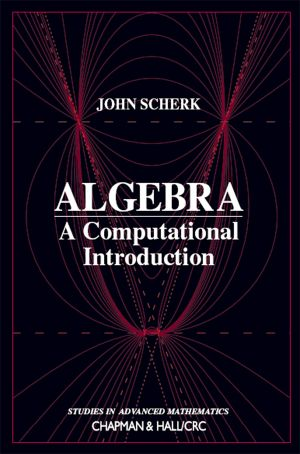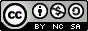# Algebra

## A Computational Introduction

by John ScherkJoin

### Book Description

This open book is an introduction to algebra for undergraduates who are interested in careers which require a strong background in mathematics. It will benefit students studying computer science and physical sciences, who plan to teach mathematics in schools, or to work in industry or finance. The book assumes that the reader has a solid background in linear algebra. For the first 12 chapters elementary operations, elementary matrices, linear independence and rank are important. In the second half of the book abstract vector spaces are used. Students will need to have experience proving results. Some acquaintance with Euclidean geometry is also desirable. In fact I have found that a course in Euclidean geometry fits together very well with the algebra in the first 12 chapters. But one can avoid the geometry in the book by simply omitting chapter 7 and the geometric parts of chapters 9 and 18.

Chapter 1
Congruences
Chapter 2
Permutations
Chapter 3
Permutation Groups
Chapter 4
Linear Groups
Chapter 5
Groups
Chapter 6
Subgroups
Chapter 7
Symmetry Groups
Chapter 8
Group Actions
Chapter 9
Counting Formulas
Chapter 10
Cosets
Chapter 11
Sylow Subgroups
Chapter 12
Simple Groups
Chapter 13
Abelian Groups
Chapter 14
Polynomial Rings
Chapter 15
Symmetric Polynomials
Chapter 16
Roots of Equations
Chapter 17
Galois Groups
Chapter 18
Quartics
Chapter 19
The General Equation of the nth Degree
Chapter 20
Chapter 21
Ruler-and-Compass Constructions

### Book Details

Title
Algebra
Subject
Science and Mathematics
Publisher
Self-publishing
Published
2009
Pages
419
Edition
1
Language
English
PDF Size
2.0 MB### Related BooksThis book studies the foundations of quantum theory through its relationship to classical physics. This idea goes back to the Copenhagen Interpretation (in the original version due to Bohr and Heisenberg), which the author relates to the mathematical formalism of operator algebras originally created by von Neumann. The book therefore includes compr...This survey of the state of the art on research in early algebra traces the evolution of a relatively new field of research and teaching practice. With its focus on the younger student, aged from about 6 years up to 12 years, this volume reveals the nature of the research that has been carried out in early algebra and how it has shaped the growth o...This carefully written textbook offers a thorough introduction to abstract algebra, covering the fundamentals of groups, rings and fields. The first two chapters present preliminary topics such as properties of the integers and equivalence relations. The author then explores the first major algebraic structure, the group, progressing as far as the ...This book develops the essential tools of linear algebra, with the goal of imparting technique alongside contextual understanding. Applications go hand-in-hand with theory, each reinforcing and explaining the other. This approach encourages students to develop not only the technical proficiency needed to go on to further study, but an appreciation ...Linear Algebra with Applications by W. Keith Nicholson, traditionally published for many years is now being released as an open educational resource. Overall, the aim of the book is to achieve a balance among computational skills, theory, and applications of linear algebra. It is a relatively advanced introduction to the ideas and techniques of ...Category Theory is one of the most abstract branches of mathematics. It is usually taught to graduate students after they have mastered several other branches of mathematics, like algebra, topology, and group theory. It might, therefore, come as a shock that the basic concepts of category theory can be explained in relatively simple terms to anybod...